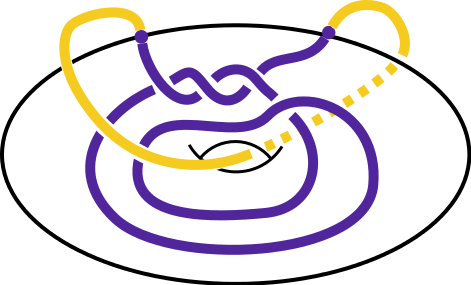High School Mathematics ContestContest Home > Contest Logo -> 2015 Contest Logo

## 2015 Contest LogoWe owe the T-shirt design and our 2015 contest logo design to Prof. Susan Abernathy of Angelo State University Math Department. Professor Abernathy graduated from the LSU mathematics department under the direction of Professor Patrick GilmerThe purple arc inside the doughnut (also known as a solid torus) is an example of a genus-1 tangle. If we close up this purple tangle, which we call $$\mathcal{D}$$, with another arc outside the solid torus by joining together their endpoints, we get a mathematical knot. Here, we've closed up our tangle with the yellow arc. A natural question to ask is: is it possible to close up this tangle and get an unknot (a knot which is really just a circle)? In this case, the answer is yes. The knot made up of the purple and yellow arcs is indeed an unknot.

Sometimes we can use algebraic objects called Kauffman bracket ideals to try to answer this question in the negative. To compute these ideals, we use the Kauffman bracket skein module (KBSM). Part of computing the Kauffman bracket ideals involves writing the tangle as a linear combination of a special basis $$\{ g_{i,\varepsilon}\}$$ for the KBSM. Here we see a formula for $$\langle \mathcal{D}, g_{i,\varepsilon}\rangle_D$$, which represents the non-zero coefficients of that linear combination.Contest organizer: Contest e-mail:   Contest web-page: Mark Davidson, phone: (225) 578-1581 contest@math.lsu.edu www.math.lsu.edu/~contest